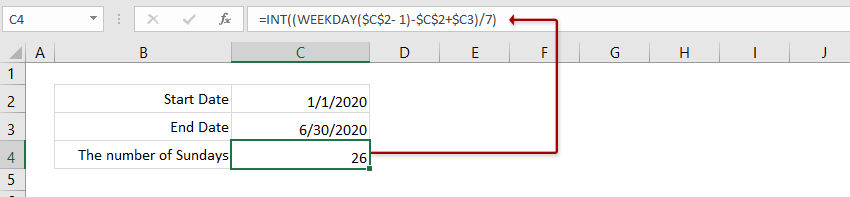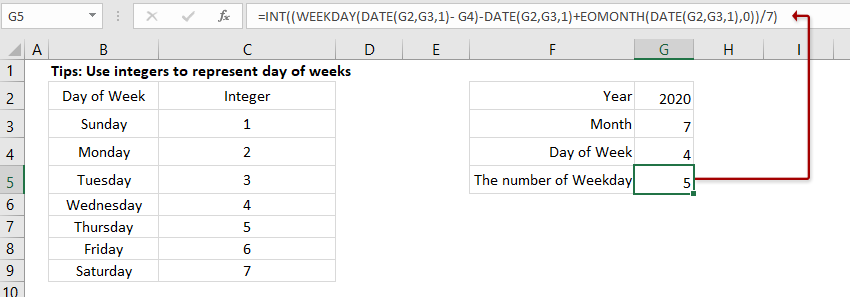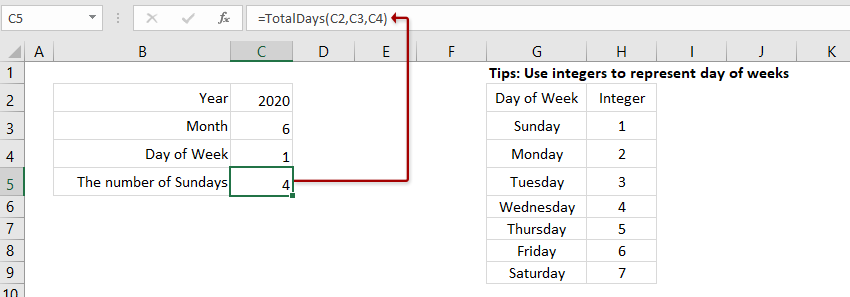# How To Count The Number Of Weekends/Weekdays Between Two Dates In Excel?

Sometimes, we need to know how many specific weekdays between two dates. For example, I have two dates: the start date is 1/1/2014 and the end date is 2/15/2014, now I want to know how many times a Sunday or a Monday or a Tuesday etc occurs in this duration. Maybe this is somewhat difficult for us, but here, I can talk about some effective methods for you.

#### Count The Number Of Specific Weekdays/Weekends Between Two Dates With Formula

Supposing, I have the following two dates, and I need to count how many Sundays between them. Select a blank cell, enter below formula, and press the Enter key. And now you will get the number of Sundays between the two dates. See screenshot:=INT((WEEKDAY(\$C\$2- 1)-\$C\$2+\$C3)/7)

Another Sample here:

=INT((WEEKDAY(\$C\$4-1)-\$C\$4+\$C\$5)/7)

Notes:

(1) In the above formula, C2 is the start date and C3 indicates the end date.

(2) In above formula, 1 stands for Sunday. And you can replace the number 1 with other numbers between 1 and 7. (1 is Sunday, 2 is Monday, 3 is Tuesday, 4 is Wednesday, 5 is Thursday, 6 is Friday and 7 is Saturday)

#### Use Formula To Count The Number Of Specific Weekday In Month

Sometimes, you may need to count the total number of a certain weekday in the given month, says count the total number of Wednesday in July, 2020. Here, I will introduce a formula to count the total number of a certain weekday in the specified month easily.

Select a blank cell, type below formula, and press the Enter key to get the counting result.=INT((WEEKDAY(DATE(G2,G3,1)- G4)-DATE(G2,G3,1)+EOMONTH(DATE(G2,G3,1),0))/7)

Notes:

(1) In above formula, G2 is the specified year, G3 is the specified month, and G4 is the specified day of week.

(2) This formula assigns integers to represent day of weeks: 1 is Sunday, 2 is Monday, 3 is Tuesday, 4 is Wednesday, 5 is Thursday, 6 is Friday, and 7 is Saturday.

#### Use User Defined Function To Count The Number Of Specific Weekdays/Weekends In A Given Month

You can also create a User Defined Function to calculate how many specific weekdays in a given year and month besides the above formula.

1. Hold down the ALT + F11 keys, and it opens the Microsoft Visual Basic for Applications window.

2. Click Insert > Module, and paste the following code in the Module Window.

Public Function TotalDays(pYear As Integer, pMonth As Integer, pDay As Integer)
Dim xindex As Integer
Dim endDate As Integerend
Date = Day(DateSerial(pYear, pMonth + 1, 0))
For xindex = 1 To endDate
If Weekday(DateSerial(pYear, pMonth, xindex)) = pDay Then
TotalDays = TotalDays + 1
End If
Next
End Function

3. Save this code and return to the worksheet, then in a blank cell, enter this formula = TotalDays(year, month, 1) . In this example, I will count how many Sundays there are in June 2020, so I can apply this formula as one of below formulas, then press Enter key, and you will get how many Sundays at once. see screenshots:=TotalDays(C2,C3,C4)

=TotalDays(2020,6,1)

Notes: This formulas use integers to represent day of weeks: 1 is Sunday, 2 is Monday, 3 is Tuesday, 4 is Wednesday, 5 is Thursday, 6 is Friday and 7 is Saturday.

Ref: https://www.extendoffice.com/documents/excel/1421-excel-count-mondays-sundays-between-two-dates.html